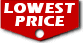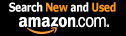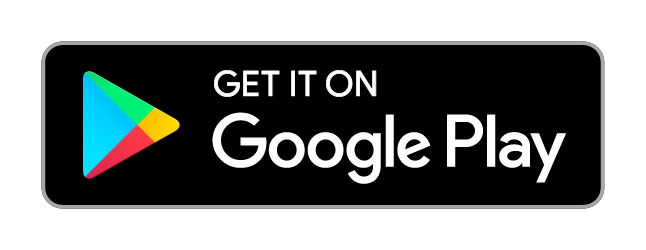# Pure Mathematics for Beginners: A Rigorous Introduction to Logic, Set Theory, Abstract Algebra, Number Theory, Real Analysis, Topology, Complex Analysis, and Linear Algebra / Steve Warner,

List Price: \$54.99
Our Price: \$54.99For Bulk orders
Quantity

Used Book Price:
/ Steve Warner,
Publisher: Get 800
Availability:
Sales Rank: 122009
 ISBN-10: 0999811754ISBN-13: 9780999811757

Similar Books
 Set Theory for Beginners: A Rigorous Introduction to Sets, Relations, Partitions, Functions, Induction, Ordinals, Cardinals, Martin's Axiom, and Stationary Sets Topology for Beginners: A Rigorous Introduction to Set Theory, Topological Spaces, Continuity, Separation, Countability, Metrizability, Compactness, ... Function Spaces, and Algebraic Topology No-Nonsense Classical Mechanics: A Student-Friendly Introduction Abstract Algebra for Beginners: A Rigorous Introduction to Groups, Rings, Fields, Vector Spaces, Modules, Substructures, Homomorphisms, Quotients, ... Group Actions, Polynomials, and Galois Theory Abstract Algebra: A Student-Friendly Approach Physics from Finance: A gentle introduction to gauge theories, fundamental interactions and fiber bundles Calculus in 5 Hours: Concepts Revealed so You Don't Have to Sit Through a Semester of Lectures No-Nonsense Quantum Mechanics: A Student-Friendly Introduction, Second Edition Machine Learning: An Applied Mathematics Introduction No-Nonsense Electrodynamics: A Student Friendly Introduction

Pure Mathematics for Beginners consists of a series of lessons in Logic, Set Theory, Abstract Algebra, Number Theory, Real Analysis, Topology, Complex Analysis, and Linear Algebra. The 16 lessons in this book cover basic through intermediate material from each of these 8 topics. In addition, all the proofwriting skills that are essential for advanced study in mathematics are covered and reviewed extensively. Pure Mathematics for Beginners is perfect for
• an introductory college course in higher mathematics.
• high school teachers working with advanced math students.
• high school and college students wishing to see the type of mathematics they would be exposed to as a math major.
The material in this pure math book includes:
• 16 lessons in 8 subject areas.
• A friendly but rigorous treatment of all the mathematics covered.
• Additional analyses before and after proofs to help students gain a deep understanding of the subject matter with the minimum amount of effort.
• A problem set after each lesson containing problems arranged by difficulty level.
A complete solution guide as a downloadable PDF file is included with purchase of this book.

Introduction
Lesson 1 - Logic: Statements and Truth
Lesson 2 - Set Theory: Sets and Subsets
Lesson 3 - Abstract Algebra: Semigroups, Monoids, and Groups
Lesson 4 - Number Theory: Ring of Integers
Lesson 5 - Real Analysis: The Complete Ordered Field of Reals
Lesson 6 - Topology: The Topology of R
Lesson 7 - Complex Analysis: The Field of Complex Numbers
Lesson 8 - Linear Algebra: Vector Spaces
Lesson 9 - Logic: Logical Arguments
Lesson 10 - Set Theory: Relations and Functions
Lesson 11 - Abstract Algebra: Structures and Homomorphisms
Lesson 12 - Number Theory: Primes, GCD, and LCM
Lesson 13 - Real Analysis: Limits and Continuity
Lesson 14 - Topology: Spaces and Homeomorphisms
Lesson 15 - Complex Analysis: Complex Valued Functions
Lesson 16 - Linear Algebra: Linear Transformations
Now you can buy Books online in USA,UK, India and more than 100 countries.
*Terms and Conditions apply
Disclaimer: All product data on this page belongs to.
No guarantees are made as to accuracy of prices and information.